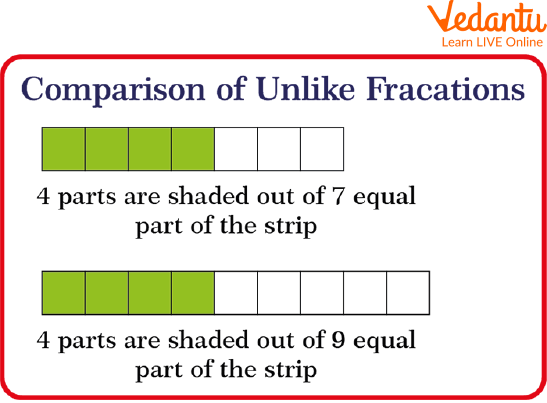Courses
Courses for Kids
Free study material
Free LIVE classes
More

# Comparison of Unlike FractionsLIVE
Join Vedantu’s FREE Mastercalss

## Overview of the Comparison of Unlike Fractions

There are several ways we use fractions unknowingly while performing our day to day activities. It is fair to say that the world would not be without fractions as we see it today. One can compare fractions with unlike denominators by finding the least common denominator, or the smallest multiple the denominators share. Then we tend to make equivalent fractions, or fractions that represent the constant part of the total. As an example $\dfrac{1}{2}, \dfrac{1}{3}, \dfrac{1}{4}, \dfrac{1}{5}$ these are unlike fractions.

## What is Fraction?

The terms used to determine the parts of a whole object are called fractions. For example, a pizza is divided into 4 pieces, so each piece of it is represented as $\dfrac{1}{4}$th of the pizza. Here 1 will be the numerator and 4 will be the denominator.

## Types of Fraction

On the basis of numerator and denominator, apart from these three main types of fractions, there are three more types of fractions namely like & unlike fractions and equivalent fractions. Hence, there are total 6 types of fractions such as:

1. Proper Fraction

2. Improper Fraction

3. Mixed Fraction

4. Like Fractions

5. Unlike Fractions

6. Equivalent Fractions

Note: The primary three fractions are defined for a single fraction however the other three fractions determine the comparison between two or more fractions.

## Unlike Fractions

Unlike fractions, fractions which have unequal denominators or different denominators. As an example $\dfrac{1}{2}, \dfrac{1}{3}, \dfrac{1}{4}, \dfrac{1}{5}$, were unlike fractions.

Simplification for such fractions is a little lengthy method since we need to factorize the denominator first and then simplify them (in case of addition and subtraction).

• Suppose, we have to add $\dfrac{1}{2}$ and $\dfrac{1}{3}$. First of all we will find the LCM of 2 and 3 which is equal to 6.

• Then we will multiply $\dfrac{1}{2}$ by 3 and $\dfrac{1}{3}$ by 2, in both the numerator and denominator.

• The fractions will become $\dfrac{3}{6}$ and $\dfrac{2}{6}$.

• Then, if $\dfrac{3}{6}$ and $\dfrac{2}{6}$ are added , we get;

• $\dfrac{3}{6}+\dfrac{2}{6}=\dfrac{5}{6}$

## Comparing Fractions

To compare unlike fractions, we change the unlike fractions to like fractions and then compare.

On comparison of two fractions with equal numerator,

$\dfrac{4}{7} \text { and } \dfrac{4}{9}$ whose numerator is the same.Comparison of unlike fractions

Since 4 shaded elements of 7 is larger than the 4 shaded elements of 9 thus, $\dfrac{4}{7}>\dfrac{4}{9}$

To compare two fractions with totally different numerators and denominators, we multiply by a number to convert them into like fractions.

## Solved Examples

Q 1. Which one is greater, $\dfrac{4}{7}$ Or $\dfrac{3}{5}$

Ans: First we convert these fractions into like fractions. To convert unlike fractions into like fractions first of all find the L.C.M. of their denominators.

L.C.M. of 7 and 5 will be $=35$

Now, On dividing the L.C.M. by the denominator of both fractions.

$35 \div 7=5$

$35 \div 5=7$

On Multiplying both the numerator and denominator with the number we get after dividing.

i.e.,

$\dfrac{4 \times 5}{7 \times 5}=\dfrac{20}{35}$

$\dfrac{3 \times 7}{5 \times 7}=\dfrac{21}{35}$

because, $\dfrac{21}{35}>\dfrac{20}{35}$ (because the denominator is the same and hence by checking the numerator, $21>20)$

So,

$\dfrac{3}{5}>\dfrac{4}{7}$

One can compare the two fractions by cross multiplication method also.

On solving the above example by cross multiplication. We will cross-multiply as follows.

$4 \times 5=20$

$3 \times 7=21$

Since, $21>20$

Therefore, $\dfrac{3}{5}>\dfrac{4}{7}$

## Practice Questions

Write the correct comparison symbol.

Q 1. $\dfrac{2}{10} \square \dfrac{2}{5}$

Ans: $\dfrac{2}{10} < \dfrac{2}{5}$

Q 2. $\dfrac{1}{10} \square \dfrac{1}{5}$

Ans: $\dfrac{1}{10} < \dfrac{1}{5}$

Q 3. $\dfrac{3}{4} \square \dfrac{4}{7}$

Ans: $\dfrac{3}{4} > \dfrac{4}{7}$

## Summary

In this Article about unlike fractions we have learnt that one can compare fractions with unlike denominators by finding the least common denominator, or the smallest multiple the denominators share. Then we tend to make equivalent fractions, or fractions that represent the constant part of the total And practice questions which will help to grasp the topic more easily. This blog shed light on fractions and how they are applied in different mathematics branches and how they impact our everyday life.

Last updated date: 19th Sep 2023
Total views: 76.8k
Views today: 1.76k

## FAQs on Comparison of Unlike Fractions

1.What are like fractions and unlike fractions?

When two fractions have the same denominator, then they are said to be like fractions. As an example, $\dfrac{3}{2}$ and $\dfrac{5}{2}$ are like fractions, and we will perform addition and subtraction operations on them. Such as:

$\dfrac{3}{2}$ + $\dfrac{5}{2}$ = $\dfrac{3+5}{2}$ = $\dfrac{8}{2}$ = 4

If two fractions have different denominators, then they are said to be unlike fractions. For example, $\dfrac{3}{2}$ and $\dfrac{4}{3}$ are improper fractions and we need to rationalize the denominators to perform addition and subtraction.

$\dfrac{(3 \times 3)}{(2 \times 3)}+\dfrac{(4 \times 2)}{(3 \times 2)}$

$=\dfrac{9}{6}+\dfrac{8}{6}$

$=\left(\dfrac{9+8}{6}\right)$

$=\dfrac{17}{6}$

2. What are the types of fractions?

The three major types of fractions are:

• Proper fraction: Fraction where numerator is lesser than the denominator.

• Improper fraction:Fraction where numerator is greater than or equal to the denominator.

• Mixed fraction: a fraction represented with its quotient and remainder.

3. What is a proper fraction? Give an example.

A proper fraction is a fraction which has its numerator value less than the denominator. For   example,$\dfrac{2}{3}$, $\dfrac{6}{7}$, $\dfrac{8}{9}$, etc. are proper fractions.